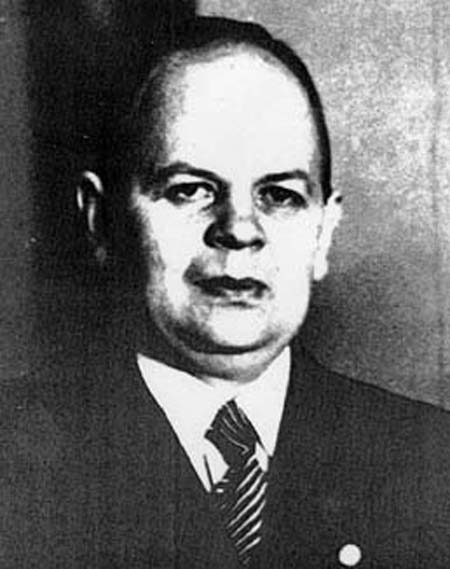A# Ackermann functionThe Ackermann function is one of the most important functions in computer science. Its most outstanding property is that it grows astonishingly fast. In fact, it gives rise to such large numbers very quickly that these numbers, called Ackermann numbers, are written in a special way known as Knuth's up-arrow notation.

The Ackermann function was discovered and studied by Wilhelm Ackermann (1896–1962) in 1928. Ackermann worked as a high-school teacher from 1927 to 1961 but was also a student of the great mathematician David Hilbert in Göttingen and, from 1953, served as an honorary professor in the university there. Together with Hilbert he published the first modern textbook on mathematical logic. The function he discovered, and that now bears his name, is the simplest example of a well-defined and total function that is also computable but not primitive recursive (PR). "Well-defined and total" means that the function is internally consistent and doesn't break any of the rules laid down to define it. "Computable" means that it can, in principle, be evaluated for all possible input values of its variables. "Primitive recursive" means that it can be computed using only for loops – repeated application of a single operation a predetermined number of times. The recursion, or feedback loop, in the Ackermann function overruns the capacity of any for loop because the number of loop repetitions isn't known in advance. Instead, this number is itself part of the computation, and grows as the calculation proceeds. The Ackermann function can only be calculated using a while loop, which keeps repeating an action until an associated test returns false. Such loops are essential when the programmer doesn't know at the outset how many times the loop will be traversed. (It's now known that everything computable can be programmed using while loops.)

The Ackermann function can be defined as follows:

A(0, n) = n + 1 for n = 0
A(m, 0) = A(m – 1, 1) for m = 1
A(m, n) = A(m – 1, A(m, n – 1)) for m, n = 1

Two positive integers, m and n, are the input and A(m, n) is the output in the form of another positive integer. The function can be programmed easily in just a few lines of code. The problem isn't the complexity of the function but the awesome rate at which it grows. For example, the innocuous-looking A(4, 2) already has 19,729 digits! The use of a powerful large-number shorthand system, such as the up-arrow notation, is indispensable as the following examples show:

A(1, n) = 2 + (n + 3) – 3
A(2, n) = 2 × (n + 3) – 3
A(3, n) = 2↑(n + 3) – 3
A(4, n) = 2↑(2↑(2↑ (...↑2))) – 3 (n + 3 twos) = 2↑↑(n + 3) – 3
A(5, n) = 2↑↑↑(n + 3) – 3 etc.

Intuitively, the Ackermann function defines generalizations of multiplication by two (iterated additions) and exponentiation with base 2 (iterated multiplications) to iterated exponentiation, iteration of this operation, and so on.

### Reference

1. Dötzel, Gunter. "A Function to End All Functions." Algorithm: Recreational Programming 2.4, 16–17 (1991).